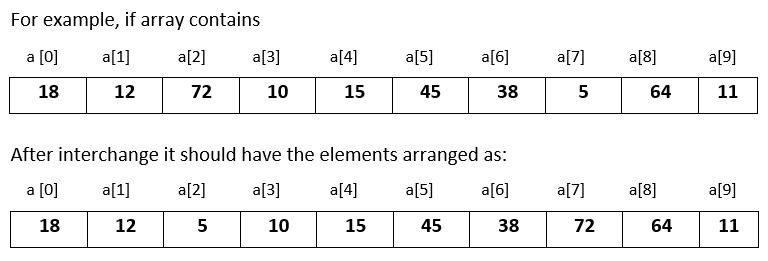# Input 10 numbers in 1d array and swap the largest and smallest number in C

###### One Dimensional Array - Question 6

In this question, we will see how to input 10 numbers in a one dimensional integer array and interchange the largest number with the smallest number within the array and print the modified array in C programming. To know more about one dimensional array click on the one dimensional array lesson.

Q6) Write a program in C to input 10 numbers in a one dimensional integer array and interchange the largest number with the smallest number within the array and print the modified array. Assume that there is only one largest and smallest number.#### Program

``````#include <stdio.h>
#include <conio.h>

int main()
{
int a, i,ln,lnp,sn,snp;

printf("Enter 10 numbers\n");
for(i=0; i<10; i++)
{
scanf("%d",&a[i]);
}

for(i=0; i<10; i++)
{
if(i==0)
{
ln=a[i];
sn=a[i];
lnp=i;
snp=i;
}
else if(a[i]>ln)
{
ln=a[i];
lnp=i;
}
else if(a[i]<sn)
{
sn=a[i];
snp=i;
}
}

a[lnp]=sn;
a[snp]=ln;

printf("\nArray after interchanging largest with the smallest\n");
for(i=0; i<10; i++)
{
printf("%d ",a[i]);
}
return 0;
}``````

#### Output

```Enter 10 numbers
18
12
72
10
15
45
38
5
64
11

Array after interchanging largest with the smallest
18 12 5 10 15 45 38 72 64 11```